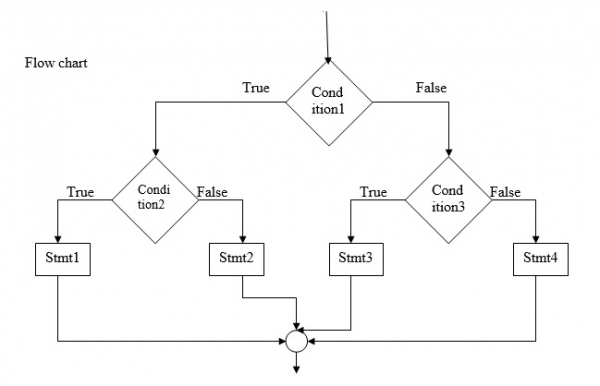# Explain Nested if-else statement in C language

A ‘nested if’ is an if statement that is the object of either if (or) an else. ‘if’ is placed inside another if (or) else.

## Syntax

Refer the syntax given below −

if (condition1){
if (condition2)
stmt1;
else
stmt2;
}
else{
if (condition3)
stmt3;
else
stmt4;
}## Example

Given below is the C program to execute Nested If Else conditional operators −

Live Demo

#include<stdio.h>
void main (){
int a,b,c,d;
printf("Enter the values of a,b,c: ");
scanf("%d,%d,%d",&a,&b,&c);
if((a>b)&&(a>c)){//Work with 4 numbers//
if(a>c){
printf("%d is the largest",a);
} else {
printf("%d is the largest",c);
}
} else {
if(b>c){
printf("%d is the largest",b);
} else {
printf("%d is the largest",c);
}
}
}

## Output

You will see the following output −

Enter the values of a,b,c: 3,5,8
8 is the largest

## Example

Following is the C program to check the number is positive or negative

Live Demo

#include <stdio.h>
int main(){
int num;
printf("Enter a number: ");
scanf ("%d ", &num);
if(num > 0){
printf("This is positive num:%d", num);
}
else if(num < 0){
printf("This is a negative num:%d",num);
} else {
printf("This is a zero:%d",num);
}
return 0;
}

## Output

You will see the following output −

Run 1: Enter a number:
23
23=This number is positive
Run 2: Enter a number:
-56
-56=This number is negative